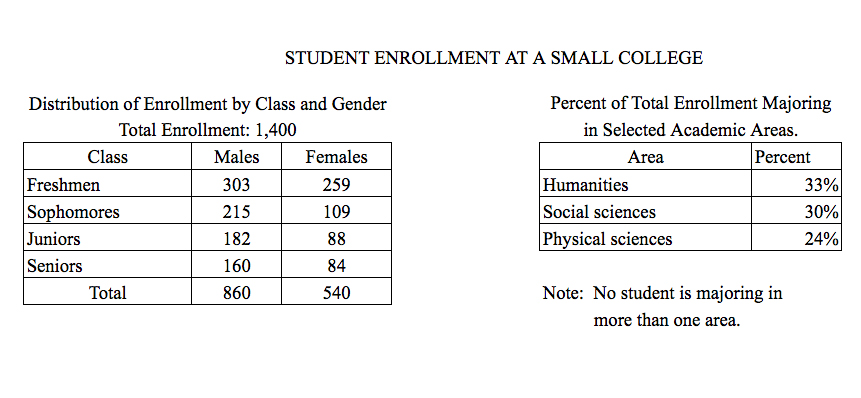# Sophomores constitute approximately what percent of the totalSophomores constitute approximately what percent of the total enrollment?

1. \$23%\$
2. \$20%\$
3. \$18%\$
4. \$15%\$
5. \$8%\$

So, you were trying to be a good test taker and practice for the GRE with PowerPrep online. Buuuut then you had some questions about the quant section—specifically question 16 of Section 6 of Practice Test 1. Those questions testing our knowledge of Graphical Methods for Describing Data can be kind of tricky, but never fear, PrepScholar has got your back!

## Survey the Question

Let’s search the problem for clues as to what it will be testing, as this will help shift our minds to think about what type of math knowledge we’ll use to solve this question. Pay attention to any words that sound math-specific and anything special about what the numbers look like, and mark them on our paper.

Looks like we’ll need to analyze two tables containing data in this question, so it likely will draw on what we’ve learned about Graphical Methods for Describing Data. Let’s keep what we’ve learned about this skill at the tip of our minds as we approach this question.

## What Do We Know?

Let’s carefully read through the question and make a list of the things that we know.

1. We want to find the percent of sophomores out of all of the students

## Develop a Plan

We want to know the percent of all of the students who are sophomores. We know that if we had the number of sophomores and the total number of students, then we could just divide these two values, multiply by \$100%\$ and have our answer.

The total number of students is given to us in the first table: \$1{,}400\$. Sophomore can be males or females, so we can get the number of sophomores by adding together the male sophomores and the females sophomores. Looks like we have a well-developed plan, so let’s finish solving this question.

## Solve the Question

Adding together the male and female sophomores, we get:

 \$\Number \of \Sophomores\$ \$=\$ \$\Male \Sophomores + \Female \Sophomores\$ \$ \$ \$ \$ \$\Number \of \Sophomores\$ \$=\$ \$215+109\$ \$ \$ \$ \$ \$\Number \of \Sophomores\$ \$=\$ \$324\$

Now we just need to divide by the total number of students to get the percent that are sophomores

 \$\Percent \of \Sophomores\$ \$=\$ \${\Number \of \Sophomores}/{\Total \Number \of \Students}·100%\$ \$ \$ \$ \$ \$\Percent \of \Sophomores\$ \$=\$ \$324/{1{,}400}·100%\$ \$ \$ \$ \$ \$\Percent \of \Sophomores\$ \$=\$ \$23%\$

Well, that wasn’t so bad after all. That problem never stood a chance, not with our knowledge about how percentages work. The correct answer is A, \$23%\$.

## What Did We Learn

To find percents, we just do the number we’re interested in divided by the total number. Here, we just had to be careful to make sure that we added together the number of male and female sophomores to get the total number of sophomores. We can be sure that the devious GRE test writers put in wrong answer choices to punish us if we forgot to add these together. Great work by us being patient and taking the time to read the full question to avoid this type of careless error.

Want more expert GRE prep? Sign up for the five-day free trial of our PrepScholar GRE Online Prep Program to access your personalized study plan with 90 interactive lessons and over 1600 GRE questions.